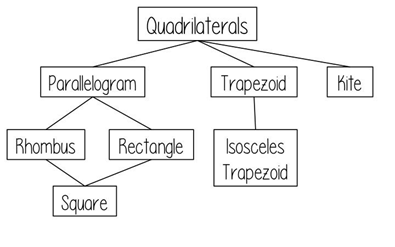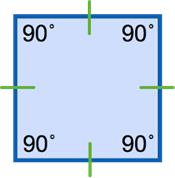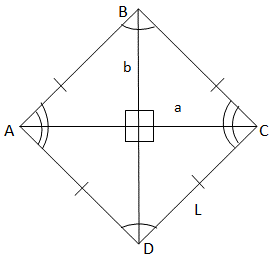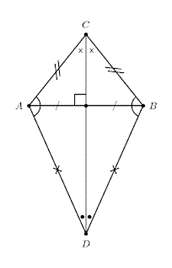Mathematics

# What are Quadrilaterals? Different types, Formulae, and Properties

1.4k views

17 December 2020

## Introduction

Polygons that have four edges and four vertices are known as Quadrilaterals. In real life, many objects resemble Quadrilaterals. A Quadrilateral has some basic properties i.e. it may be a regular or irregular shape, its interior angles sum up to 360°. Lets see the types of quadrilaterals with their properties and some examples.

## What are Quadrilaterals? - PDF

If you ever want to read it again as many times as you want, here is a downloadable PDF to explore more.

All the Quadrilaterals might have the basic properties but differ in some kind. All types of Quadrilaterals have their own unique properties.

They are as follows:1. Square
2. Rectangle
3. Parallelogram
4. Trapezium
5. Rhombus
6. Kite### Formulas of a Square

• Area of a Square = side $$\times$$ side, the side length of the square multiplied with the side itself.
• Perimeter of a Square = 4 $$\times$$ side, four times the side length.
• Diagonal of a Square = $$a\sqrt{2}$$, where a is the side of the square.

### Properties of a square

• All interior angles are 90°.
• All sides of a square are equal in length.
• The diagonals of a square are also equal in length.
• The diagonals make two equal isosceles triangles. The intersection of Diagonals forms a perpendicular angle.
• Opposites sides of the squares are always parallel.

Example:

Chessboard### Formulas of a Rectangle

• Area of the rectangle = Length × Breadth
• Perimeter of the Rectangle = Length + Length + Breadth + Breadth>

= 2 $$\times$$ Length + 2 × Breadth

Perimeter of the Rectangle = 2 $$\times$$ ( Length + Breadth)

• Diagonal of the Rectangle $$= d2 = \sqrt{(l^2 + b^2)},$$ where l and b are the Rectangle’s length and breadth.

### Properties of a Rectangle

• The interior angles of a rectangle are 90°.
• The opposite sides of a Rectangle are parallel and equal.
• The diagonal is the line that joins the opposite vertices.
• The diagonal of the Rectangle creates two pairs of scalene triangles.

Example:

TVs, Mobile phones, Carpets### Formulas of a parallelogram

• Area of the Parallelogram = b $$\times$$ h, where b is the base of the parallelogram base and h be the altitude of the parallelogram.
• Perimeter of the perimeter = 2 $$\times$$ (base + side length)

### Properties of a parallelogram

• It has two pairs of equal opposite sides parallel to each other.
• Sum of their interior angles are 360°.
• Their opposite angles are equal.
• Their diagonals bisect each other.

Examples:

tangrams### Formulas of a trapezium

• Area of the trapezium = ½ × h (a + b), where h is the altitude and a and b are the two base lengths.
• Perimeter of the trapezium = sum of all sides of the trapezium.
• Median of the trapezium is the line segment, which is halfway between the two bases. It is called the midsegment.

M = (a + b)/2

### Properties of a trapezium

• Trapeziums have two opposite unequal parallel sides.
• The interior angles of a trapezium add up to 360°.### Formulas for rhombus:

• Area of the rhombus = altitude $$\times$$ side of the rhombus

Another way to calculate the area of the rhombus be divide the product of the two diagonals

Area $$= P \frac{Q}{2}$$

• Perimeter of the rhombus $$= 4 \times$$ side of the rhombus.

### Properties of a rhombus

• All four sides are equal.
• The diagonals intersect at 90°.### Formulas for kites

• Perimeter of a kite $$= 2 \times$$ (a + b)
• Area of a kite $$= h_1 × h_2$$

### Properties of a kite

• The diagonals intersect at 90°.
• The two adjacent sides are equal to each other.
• The diagonals bisect each other.

## Summary

This blog talks about the different quadrilaterals, their properties, and formulae to determine their area and perimeter. It discusses in length and covers all the basics of quadrilaterals.

Written by Suganya Balaji, Cuemath Teacher

Cuemath, a student-friendly mathematics and coding platform, conducts regular Online Live Classes for academics and skill-development, and their Mental Math App, on both iOS and Android, is a one-stop solution for kids to develop multiple skills. Understand the Cuemath Fee structure and sign up for a free trial.

## How to find the Area of a parallelogram?

Area of the Parallelogram $$= b \times h$$

## What is the formula for Area of a square?

Area of a square $$= a × a$$

a = Length of side

## What is the formula for Area of a rectangle?

Area of a rectangle $$= w \times h$$

W = width

h = height

## What is the formula for Area of a parallelogram?

Area of a parallelogram $$= b \times h$$

b = base

h = vertical height

## What is the formula for Area of a trapezium?

Area of a trapezium $$= ½ (a + b) \times h$$

h = vertical height

## What is the formula for Area of a rhombus?

Area of a rhombus $$= P \times \frac{Q}{2}$$

## What is the formula for Perimeter of a square?

“The perimeter of any closed geometrical object is defined as the distance around that object.”

Perimeter of a square $$= 4 \times a$$

## What is the formula for Perimeter of a rectangle?

The perimeter of a rectangle is defined as the sum of all the sides of a rectangle.

Perimeter of a rectangle $$= 2 \times (l + b)$$

## What is the formula for Perimeter of a parallelogram?

The sum of the sides of the parallelogram is the perimeter of the parallelogram.

## What is the formula for Perimeter of a trapezium?

The sum of the all sides of the trapezium is called the perimeter of the trapezium.

## What is the formula for Perimeter of a rhombus?

The sum of all the sides of the rhombus is the perimeter of the rhombus.

Perimeter of a rhombus $$= 4 \times a$$

## What is the formula for Perimeter of a kite?

A kite has two pairs of equal sides. Total distance around the outside is called the perimeter of a kite.